## Laws of the Land

Shmoop has a dream, a dream where all triangles can live in a place of peace and harmony together. Where triangles of all types can coexist, no matter their angle measurements or side lengths. Where every triangle will be free to explore the wonders of trigonometry, and all will be able to truly find themselves in this safe haven.

Thankfully, this dream is about to become reality. After all, just because some triangles are right doesn't mean that other ones are wrong. Trigonometry doesn't discriminate and neither should we. And so every triangle—great or small, obtuse or acute, isosceles or scalene—can enjoy the wonders of trigonometry.

To maintain order in our newfound triangle paradise, however, we should set a few ground rules. With these laws of the land in place, we'll be able to solve any triangle for any side length or angle measure. And it'll make sure those greedy right triangles don't hog all the trig.

• ### Law of Sines

The Law of Sines can be used to find the angles and side lengths of triangles that aren't right triangles. It has little to do with crop circles, aliens, or Mel Gibson.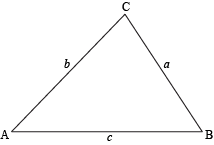The triangle above represents any non-right triangle. The Law of Sines says that for such a triangle: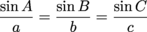We can prove it, too. All we have to do is cut that triangle in half.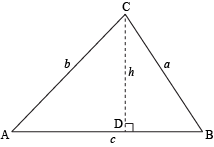Using the trig ratios we learned, we can find the sine of angles A and B for the two right triangles we made.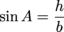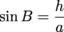Isolate for the altitude h and then set the two equations equal to each other.

b sin A = h
a sin B = h
b sin A = a sin B

Dividing both sides by ab will give us our Law of Sines.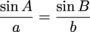If draw different altitudes and repeat all that business, we'll end up with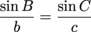and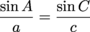. Solid proof, we think. Now that we know it's true, we can start putting it to use.

### Sample Problem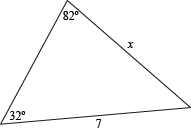We'll start by finding the value of x. The Law of Sines is still fresh in our minds.We'll set A to 82° and B to 32°. That means a is 7 and b is x.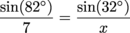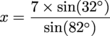x ≈ 3.7

Simple enough.

The Law of Sines is so useful that with it, we're unstoppable. We can solve triangles, which means finding every side length and every angle measurement.

### Sample Problem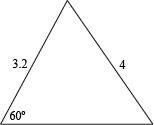Solving this triangle should be pretty easy. We'll start with the Law of Sines.If A is 60°, then a is 4. The only other side we have is 3.2, so that'll be b.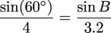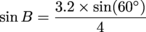We want B, not sin B. If we take the inverse sine of each side, we get: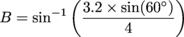B ≈ 43.9°

Wonderful. Now we have two out of three angles and two out of three sides. Knowing that all the angles in a triangle add up to 180° allows us to find the last angle.

A + B + C = 180°

Substitute in the angles we know.

60° + 43.9° + C = 180°

Solve for the remaining angle.

C = 180° – (60° + 43.9°)
C = 76.1°

One side to go. We can use either the ratio between sin(60°) and 4 or sin(43.9°) and 3.2.Substitute in what we know. We're looking for the last side, c.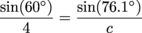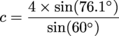c ≈ 4.5

Double-check. Do we know all the angles and all the sides?

A = 60°; B = 43.9°; C = 76.1°
a = 4; b = 3.2; c = 4.5

Yep. We solved that triangle real good.

• ### Law of Cosines

The Law of Sines can be very useful, but there are cases where it won't do. Don't get us wrong. It's taken us this far and we owe it some gratitude. But no man is an island, and the Law of Sines can't do it all alone. Take this triangle, for example.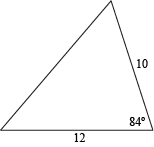Any way we try, the Law of Sines just won't cut it. Luckily, we have another law that we can use. Since this second law cooperates well with the Law of Sines, we'll call it the Law of Cosines.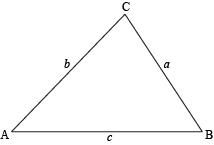For any triangle, represented by the triangle above, the Law of Cosines says that the following are true:

a2 = b2 + c2 – 2bc cos A
b2 = a2 + c2 – 2ac cos B
c2 = a2 + b2 – 2ab cos C

These formulas will help us when the Law of Sines can't, but before we use 'em, we have to prove 'em.We'll start off with a slightly altered Pythagorean Theorem. Let AD = x, which means that DB = cx. In this case, a and b are the hypotenuses.

 (c – x)2 + h2 = a2 Apply Pythagorean Theorem to ΔCDB c2 – 2cx + x2 + h2 = a2 Multiplying out (c – x)2 x2 + h2 = b2 Apply Pythagorean Theorem to ΔADC c2 – 2cx + b2 = a2 Substitute in b2 for x2 + h2 cos A = x⁄b Definition of cos A b cos A = x Rearrange (multiply both sides by b) c2 – 2bc cos A + b2 = a2 Substitute in b cos A for x a2 = b2 + c2 – 2bc cos A Rearrange, thanks to the commutative property

We're good to go. Bring it on, triangles.

### Sample ProblemNow we can solve this triangle. Let the only unknown side be a, ∠A = 84°, b = 10, and c = 12. We'll start with our fresh new law.

a2 = b2 + c2 – 2bc cos A

Fill it up.

a2 = (10)2 + (12)2 – 2(10)(12) cos(84°)
a2 = 100 + 144 – 240 cos(84°)
a2 = 244 – 25.1
a2 = 218.9
a ≈ 14.8

Great. If we wanted to solve the entire triangle, we could find the remaining angles using either the Law of Sines or the Law of Cosines. Hooray for freedom.

The Law of Sines is also useless if we have a triangle without any known angles.

### Sample Problem

What are the measures of all the angles in the following triangle?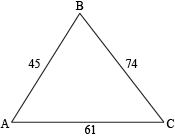With this triangle, the Law of Sines wouldn't amount to squat. Zip. Zero. Nada. Thank goodness we have the Law of Cosines to help us out.

a2 = b2 + c2 – 2bc cos A

Hold up. If we plug in all the sides now, moving the terms around will be more uncomfortable than an elephant in a broom closet. Isolating our variable will make things easier for us in the long run. When we do, we end up with this piece of work: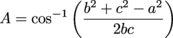Now we can substitute our values without breaking a sweat.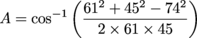All right. Time to pull out the abacus. On second thought, a calculator is probably a better idea.

A ≈ 87.2°

Nice job. Since we have an angle, we can choose whether to use the Law of Cosines or the Law of Sines to solve for the other two angles. The Law of Sines is probably easier, but that's not what this chapter's all about.

b2 = a2 + c2 – 2ac cos B

Much like the Power Rangers, it's morphin' time!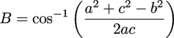Now we can plug in our side lengths.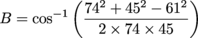B ≈ 55.4°

Wonderfully done. For the last angle, we have not one, not two, but three different options.

1. We could use the Law of Cosines.
2. We could use the Law of Sines.
3. We could use the fact that all angles in a triangle add up to 180°.

We've done them all, and they'll all give us the same answer. Just to practice, we'll give the Law of Cosines one more go.

c2 = a2 + b2 – 2ab cos C

We're still looking for an angle. Let's act like it.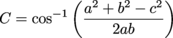Replace the letters with numbers. This is math, after all.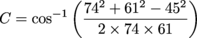C ≈ 37.4°

The Law of Cosines, while very useful, is also very jumbled. All those letters and numbers and exponents and pluses and minuses…it's easy to get confused. The Law of Sines on the other hand, is pretty chill. That's why with the Law of Cosines, it's best to cosign with some other formulas so that things don't get too muddled. Cosine…cosign…get it?